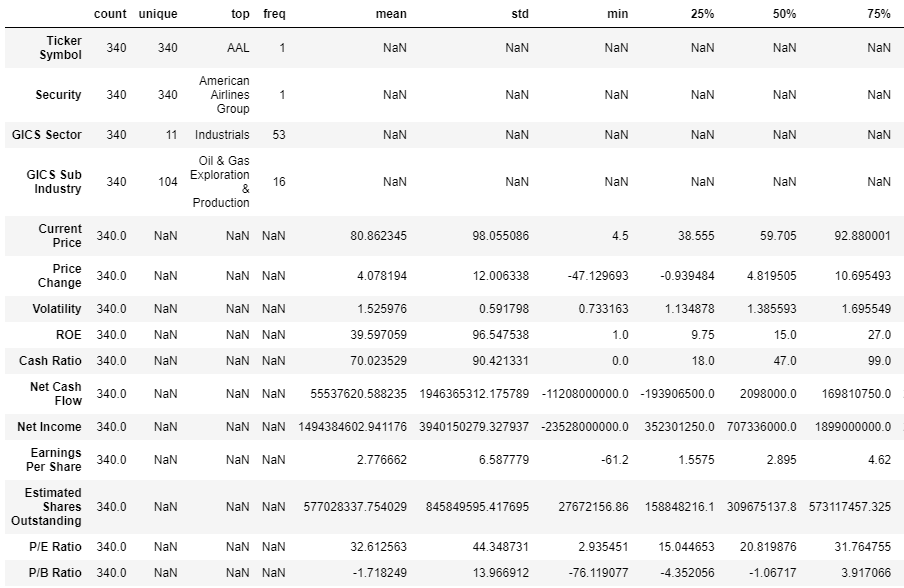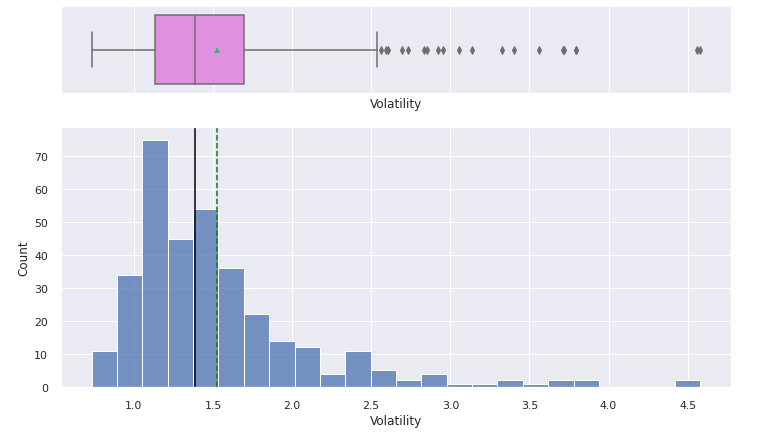top of page
Search

Updated: Apr 18

### Context

The stock market has consistently proven to be a good place to invest in and save for the future. There are a lot of compelling reasons to invest in stocks. It can help in fighting inflation, create wealth, and also provides some tax benefits. Good steady returns on investments over a long period of time can also grow a lot more than seems possible. Also, thanks to the power of compound interest, the earlier one starts investing, the larger the corpus one can have for retirement. Overall, investing in stocks can help meet life's financial aspirations.

It is important to maintain a diversified portfolio when investing in stocks in order to maximise earnings under any market condition. Having a diversified portfolio tends to yield higher returns and face lower risk by tempering potential losses when the market is down. It is often easy to get lost in a sea of financial metrics to analyze while determining the worth of a stock, and doing the same for a multitude of stocks to identify the right picks for an individual can be a tedious task. By doing a cluster analysis, one can identify stocks that exhibit similar characteristics and ones which exhibit minimum correlation. This will help investors better analyze stocks across different market segments and help protect against risks that could make the portfolio vulnerable to losses.

### Objective

Trade&Ahead is a financial consultancy firm who provide their customers with personalized investment strategies. They have hired you as a Data Scientist and provided you with data comprising stock price and some financial indicators for a few companies listed under the New York Stock Exchange. They have assigned you the tasks of analyzing the data, grouping the stocks based on the attributes provided, and sharing insights about the characteristics of each group.

### Data Dictionary

• Ticker Symbol: An abbreviation used to uniquely identify publicly traded shares of a particular stock on a particular stock market

• Company: Name of the company

• GICS Sector: The specific economic sector assigned to a company by the Global Industry Classification Standard (GICS) that best defines its business operations

• GICS Sub Industry: The specific sub-industry group assigned to a company by the Global Industry Classification Standard (GICS) that best defines its business operations

• Current Price: Current stock price in dollars

• Price Change: Percentage change in the stock price in 13 weeks

• Volatility: Standard deviation of the stock price over the past 13 weeks

• ROE: A measure of financial performance calculated by dividing net income by shareholders' equity (shareholders' equity is equal to a company's assets minus its debt)

• Cash Ratio: The ratio of a company's total reserves of cash and cash equivalents to its total current liabilities

• Net Cash Flow: The difference between a company's cash inflows and outflows (in dollars)

• Net Income: Revenues minus expenses, interest, and taxes (in dollars)

• Earnings Per Share: Company's net profit divided by the number of common shares it has outstanding (in dollars)

• Estimated Shares Outstanding: Company's stock currently held by all its shareholders

• P/E Ratio: Ratio of the company's current stock price to the earnings per share

• P/B Ratio: Ratio of the company's stock price per share by its book value per share (book value of a company is the net difference between that company's total assets and total liabilities)

Let's start coding! Importing necessary libraries

```# Libraries to help with reading and manipulating data
import numpy as np
import pandas as pd

# Libraries to help with data visualization
import matplotlib.pyplot as plt
import seaborn as sns

sns.set_theme(style='darkgrid')

# Removes the limit for the number of displayed columns
pd.set_option("display.max_columns", None)
# Sets the limit for the number of displayed rows
pd.set_option("display.max_rows", 200)

# to scale the data using z-score
from sklearn.preprocessing import StandardScaler

#to compute distances
from scipy.spatial.distance import cdist, pdist

# to perform k-means clustering and compute silhouette scores
from sklearn.cluster import KMeans
from sklearn.metrics import silhouette_score

# to visualize the elbow curve and silhouette scores
from yellowbrick.cluster import KElbowVisualizer, SilhouetteVisualizer

# to perform hierarchical clustering, compute cophenetic correlation, and create dendrograms
from sklearn.cluster import AgglomerativeClustering
from scipy.cluster.hierarchy import dendrogram, linkage, cophenet

# to suppress warnings
import warnings
warnings.filterwarnings("ignore")```
```## Complete the code to import the data
```# checking shape of the data
print(data.shape)
print(f"There are {'340'} rows and {'15'} columns.") ## Complete the code to get the shape of data```

Output:

(340, 15) There are 340 rows and 15 columns.

```# let's view a sample of the data
data.sample(n=10, random_state=1)```

Output:```# checking the column names and datatypes
data.info() ```

output:```# copying the data to another variable to avoid any changes to original data
df = data.copy()```
```# checking for duplicate values
df."--" ## Complete the code to get total number of duplicate values```
```# checking for missing values in the data
df."--" ## Complete the code to check the missing values in the data```

Output:`df.describe(include='all').T`

Output:### Univariate analysis

```# function to plot a boxplot and a histogram along the same scale.
def histogram_boxplot(df, feature, figsize=(12, 7), kde=False, bins=None):
"""
Boxplot and histogram combined

data: dataframe
feature: dataframe column
figsize: size of figure (default (12,7))
kde: whether to the show density curve (default False)
bins: number of bins for histogram (default None)
"""
f2, (ax_box2, ax_hist2) = plt.subplots(
nrows=2,  # Number of rows of the subplot grid= 2
sharex=True,  # x-axis will be shared among all subplots
gridspec_kw={"height_ratios": (0.25, 0.75)},
figsize=figsize,
)  # creating the 2 subplots
sns.boxplot(
data=df, x=feature, ax=ax_box2, showmeans=True, color="violet"
)  # boxplot will be created and a star will indicate the mean value of the column
sns.histplot(
data=df, x=feature, kde=kde, ax=ax_hist2, bins=bins, palette="winter"
) if bins else sns.histplot(
data=df, x=feature, kde=kde, ax=ax_hist2
)  # For histogram
ax_hist2.axvline(
df[feature].mean(), color="green", linestyle="--"
)  # Add mean to the histogram
ax_hist2.axvline(
df[feature].median(), color="black", linestyle="-"
)  # Add median to the histogram```

Current Price

`histogram_boxplot(df, 'Current Price')`

Output:Price Change

`histogram_boxplot(df,'--')  ## Complete the code to create histogram_boxplot for 'Price Change'`

Output:Volatility

`histogram_boxplot(df, '--')  ## Complete the code to create histogram_boxplot for 'Volatility'`

output:`histogram_boxplot(df,'--')  ## Complete the code to create histogram_boxplot for 'ROE'`

Output:Cash Ratio

`histogram_boxplot(df, '--')  ## Complete the code to create histogram_boxplot for 'Cash Ratio'`

Output:### Bivariate Analysis

```# correlation check
plt.figure(figsize=(15, 7))
sns.heatmap(
df.corr(), annot=True, vmin=-1, vmax=1, fmt=".2f", cmap="Spectral"
)
plt.show()```

Output:Realcode4you expert and professionals has more experience in data analysis related projects and assignments. Our expert do many project which is related to industries data. Here you get code without any plagiarism with full privacy. To get help with realcode4you you can send your requirement details at:

realcode4you@gmail.com